Next: Rotational kinetic energy Up: Rigid body rotation Previous: Fundamental equations

# Moment of inertia tensor

Consider a rigid body rotating with fixed angular velocity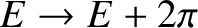about an axis that passes through the origin. (See Figure 8.1.) Let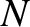be the position vector of the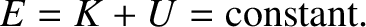th mass element, whose mass is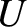. We expect this position vector to precess about the axis of rotation (which is parallel to) with angular velocity. It, therefore, follows from Section A.7 that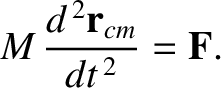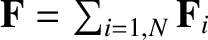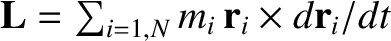(8.4)

Thus, Equation (8.4) specifies the velocity,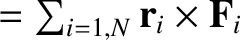, of each mass element as the body rotates with fixed angular velocityabout an axis passing through the origin.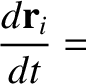The total angular momentum of the body (about the origin) is written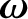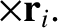(8.5)

where use has been made of Equation (8.4), and some standard vector identities. (See Section A.4.) The preceding formula can be written as a matrix equation of the form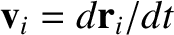(8.6)

where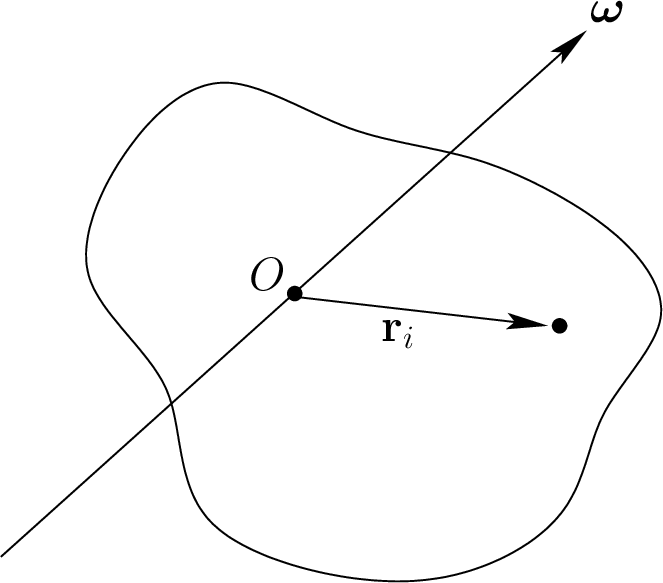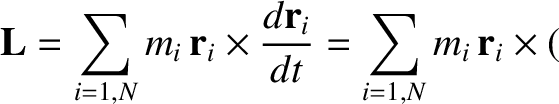(8.7)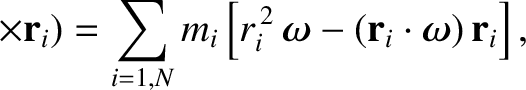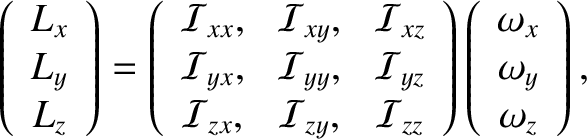(8.8)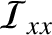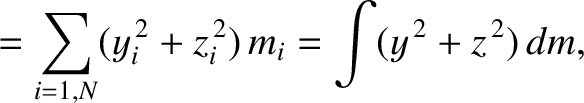(8.9)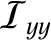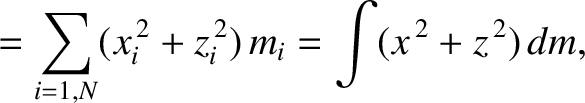(8.10)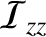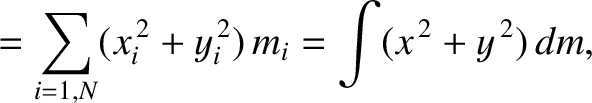(8.11) and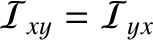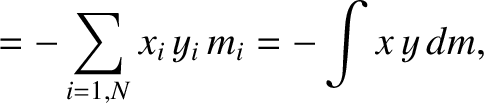(8.12)

Here,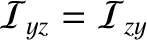is called the moment of inertia about the-axis,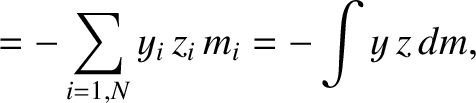the moment of inertia about the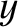-axis,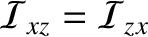the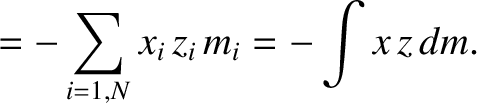product of inertia,theproduct of inertia, and so on. The matrix of the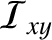values is known as the moment of inertia tensor. Each component of the moment of inertia tensor can be written as either a sum over separate mass elements, or as an integral over infinitesimal mass elements. In the integrals,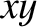, where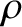is the mass density, and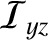a volume element. Equation (8.6) can be written more succinctly as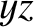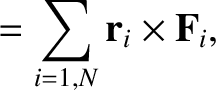(8.13)

Here, it is understood that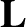andare both column vectors, and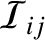is the matrix of thevalues. Note thatis a real symmetric matrix; that is,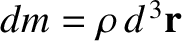and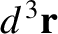.

In general, the angular momentum vector,, obtained from Equation (8.13), points in a different direction to the angular velocity vector,. In other words,is generally not parallel to.

Finally, although the preceding results were obtained assuming a fixed angular velocity they remain valid, at each instant in time, if the angular velocity varies.Next: Rotational kinetic energy Up: Rigid body rotation Previous: Fundamental equations
Richard Fitzpatrick 2016-03-31